1. ## загадка

You should put such mathematical signs between 1 1 1 so that 1 1 1 = 6

any ideaReply With Quote

2. ## Re: загадкаOriginally Posted by Mordan
You should put such mathematical signs between 1 1 1 so that 1 1 1 = 6

any idea
(1+1+1)!

I don't know if it's OK because the factorial sign is after and not between.Reply With Quote

3. ## Re: загадкаOriginally Posted by FriendyOriginally Posted by Mordan
You should put such mathematical signs between 1 1 1 so that 1 1 1 = 6

any idea
(1+1+1)!

I don't know if it's OK because the factorial sign is after and not between.
That's perfectly acceptable. It's just 3!.Reply With Quote

4. Perhaps we should write some three signs which are similar with S . / and get the word SiX SIX=6Reply With Quote

5. I think we're talking about maths here, not doodling :P.

N.B.: to doodle (int. v.) - to draw casually, often absent-mindedly.Reply With Quote

6. what does the word mean?

no - I means math, not doodling...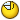Reply With Quote

7. Mathematics. "Математик", isn't it?Reply With Quote

8. Mathema...and then tics? That you did say?
Is it vegetable or mineral?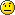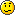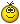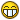I'm kidding you - oh come on you dont think I really have no idea what the math is?Reply With Quote

9. Heh, you just might not have known the short form "maths".Reply With Quote

10. No actually I know.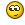I forgot the letter sReply With Quote

11. ## Re: загадкаOriginally Posted by Mordan
You should put such mathematical signs between 1 1 1 so that 1 1 1 = 6

any idea
Must I use all digits?
Can I use: +, -, x, / (divided by) several times?
Can I use square root?Reply With Quote

12. ## Re: загадкаOriginally Posted by BladeStuckinOriginally Posted by Mordan
You should put such mathematical signs between 1 1 1 so that 1 1 1 = 6

any idea
Must I use all digits?
Can I use: +, -, x, / (divided by) several times?
Can I use square root?

! is the 'factoral' sign

2! = 2x1
3! = 3x2x1
4! = 4x3x2x1

(1+1+1)! = 3! = 3x2x1 = 6Reply With Quote

13. ## Re: загадкаOriginally Posted by TATY

! is the 'factoral' sign

2! = 2+1
3! = 3+2+1
4! = 4+3+2+1

(1+1+1)! = 3! = 3+2+1 = 6
2! = 2 x 1
3! = 3 x 2 x 1
4! = 4 x 3 x 2 x 1

Someone wasn't paying attention in 'maths'.Reply With Quote

14. Typo!Reply With Quote

15.Originally Posted by TATY
Typo!
утро!Reply With Quote

####Posting Permissions

• You may not post new threads
• You may not post replies
• You may not post attachments
• You may not edit your posts
•